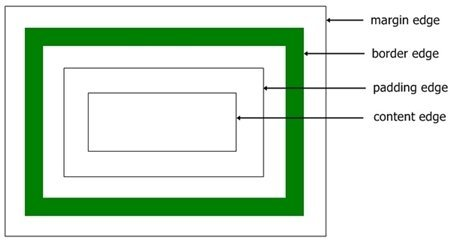CSS Dimensions

« Previous Tutorial Next Tutorial »

All HTML elements in a box model are represented as rectangular boxes. The dimensions of the box model are calculated by using the height and width of the content area that gets applied to the element.

Each box is associated with a content area and many optional areas, such as padding, border, and margin.

The size of each area is specified by using the box model dimensions shown in the following figure:In the above figure, the perimeter of the content, padding, border, and margin is called an edge. Each box in the box model have four edges, describes in the table given below:

Edge Description
content edge Surrounds a rectangle specified by the width and height of the box. This is also called the inner edge. The four content edges represent the content area of the box.
border edge Surrounds the border of the box. If the border has 0 width, the border edge becomes a padding edge. The four edges represent the border area of the box.
margin edge Surrounds the margin of the box. If the border has 0 width, the margin edge becomes a border edge. The margin edge is also called as outer edge. The four border edges represent the margin area of the box.

CSS Dimension Properties

The table given here lists all the CSS Dimension Properties:

Property Possible Values
width auto
length
%
inherit
height auto
length
%
inherit
min-width length
%
inherit
max-width none
length
%
inherit
min-height length
%
inherit
max-height none
length
%
inherit

To learn all the above properties in detail, then refer CSS Width and Height Properties

CSS Dimensions Example

You can set HTML Elements height, width, max-height, max-width, min-height, min-width as per your requirement using CSS Dimensions.

To learn more about CSS width and height property to set box dimensions, refer CSS Width and Height tutorial.

Let's look at the following example, shows how to set box dimensions using CSS:

<!DOCTYPE html>
<html>
<title>CSS Dimensions Example</title>
<body>

<p style="height:50px;background-color:green;color:white;">
You can set HTML Elements Dimensions:
</p>
<p style="max-width:300px;background-color:red;color:white;">
using height, width, max-height, max-width, min-height, min-width
</p>
<p style="width:100px;background-color:blue;color:white;">
</p>
<p style="min-height:100px;background-color:black;color:white;">
using CSS Dimensions
</p>

</body>
</html>

Here is the sample output produced by the above CSS dimensions example code:CSS Online Test

« Previous Tutorial Next Tutorial »